## ICSE Class 9 Physics Sample Question Paper 5 with Answers

Section-1
(Attempt all questions from this section)

Question 1.
(a) How will you express the result of a measurement of a physical quantity ?
(b) What do you mean by the following terms :
(i) Least count of an instrument
(ii) Vernier constant ?
(c) State the parameters on which time period of a simple pendulum does not depend.
(d) A vernier scale has 50 divisions which coincide with 49 divisions of the main scale. The main scale is graduated in 1/2 mm steps. What is the least count of the vernier ?
(e) Calculate the length of a second’s pendulum at a place where acceleration due to gravity is 9.8 m/s2.
(a) Result of a measurement of a physical quantity is expressed by a number of numerical measure accompanied by a unit. Thus, measurement of a physical quantity (Q) = Numerical measure (n) x unit (μ)

(b) (i) Least count of an instrument means the least or smallest measurement which can be measured by the instrument accurately.
(ii) Vernier constant is equal to the difference between the values of one main scale division and one vernier scale division.

(c) The period of oscillation does not depend on :
(1) mass of the material and shape of the bob
(2) amplitude of oscillation.
(a) Result of a measurement of a physical quantity is expressed by a number of numerical measure accompanied by a unit. Thus, measurement of a physical quantity (Q) = Numerical measure (n) x unit (u)(b) (i) Least count of an instrument means the least or smallest measurement which can be measured by the instrument accurately.
(ii) Vernier constant is equal to the difference between the values of one main scale division and one vernier scale division.

(c) The period of oscillation does not depend on :
(1) mass of the material and shape of the bob
(2) amplitude of oscillation.Question 2.
(a) An insect is crawling on a cycle rim of radius r. What will be the distance and displacement of the insect
(i) in half revolution, and
(ii) in one complete revolution?
(b) Fill in the blanks :
(i) The velocity-time graph of a particle in uniform motion is a straight line ………… to the time axis.
(ii) The time-distance graph of a particle in uniform motion is a straight line ………… to the time axis.
(c) Sketch the velocity-time graph for an object moving with
(i) uniform velocity
(ii) uniform acceleration.
(d) Comment on the type of motion represented by the displacement-time graph shown in the given figure. What conclusions can we draw from this graph ?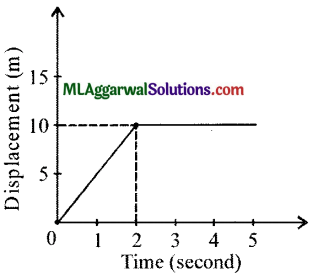(e) A particle moves 8 m due east and then through 6 m due north.
(i) How much is the net distance moved ?
(ii) What is the magnitude of the net displacement ?
(a) (i) In half revolution, distance travelled by the insect will be equal to the circumference of the half circular rim, i.e., nr and displacement will be the shortest distance between initial and final positions, i.e., 2r.(ii) In complete revolution, distance travelled by the insect will be the circumference of the rim, 2nr and displacement will be zero.(b) (i) Parallel
(ii) Inclined

(c) (i) For uniform velocity
(ii) For uniform acceleration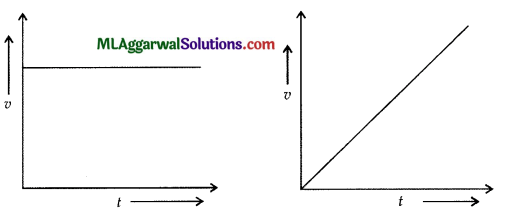(d) The particle here moves with a uniform velocity from t = 0 to t = 2s represented by an inclined line. Then the line becomes parallel showing no displacement. Therefore, it remains at rest.
The slope of the graph from f = 0tof = 2sis given by :
$$\text { Slope }=\frac{10-0}{2-0}=5$$
This represents the velocity of the particle. Thus, the particle has a uniform velocity of 5 ms-1 from t = 0 to t = 2 s. After that the particle remains at rest.

(e) The particle starts from 0. It moves through 8 m due east to reach A and then through 5 m due north to reach B.
(i) Total distance moved is8m + 6m = 14m
(ii) Magnitude of the net displacement is OB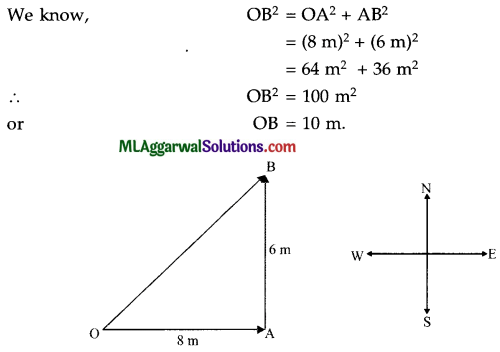Question 3.
(a) Why does a long jump runner come running before taking a leap ?
(c) What would be the difference in weight, in any, of one kilogram of cotton and one kilogram of iron, if both are weighed in vacuum ?
(d) What is the function of the gravity bulb in a hydrometer ?
(e) The portion of a wooden block, floating inside water, measures 50 cm x 50 cm x 50 cm. Find the magnitude of the buoyant force acting on the block.
(a) By running, the runner’s body acquires an ‘inertia of motion’ which tends to keep the runner moving forward. He therefore, achieves a longer jump compared to what he would have got had he taken a jump from a standing ‘at rest’ position.

(b) The use of broad base in dams decreases the pressure of the weight of water on the base. This helps the base, and hence the dam built on it, to stay intact.

(c) There would be no difference in the weights of cotton and iron in vacuum. This is because, in vacuum, there is no upthrust at all on any object. Thus, objects of equal mass, irrespective of their volume, have equal weight in vacuum.(d) The gravity bulb of a hydrometer is loaded with iron balls or pitch. This results in a lowering of the C.G. of the hydrometer as a whole. This lowering of the C.G. in turn helps the hydrometer to float in a stable, upright position when it is immersed in a liquid.

(e) The buoyant force = Weight of water displaced
= Volume of water displaced x Density of water x g
= (50 x 50 x 50 x 10-5 m3) x (1000 kg/m3) x 9.8 ms-2
= 125 x 9.8 kg ms-2
= 1225 N.

Question 4.
(a) Name four green house gases.
(b) Deep pond of water has its top layer frozen during winter. State the expected temperature of water layer (i) just in contact with ice, and (ii) at the bottom of the pond.
(c) What is the number of images formed when an object is placed between two parallel plane mirrors ? Why do the distant images appear fainter ?
(d) The speed of propagation of sound waves, in a gaseous medium equals 340 ms-1. If the frequency of these waves is 1700 Hz, find the wavelength.
(e) The upper blood pressure of a patient is 150 cm of Hg whereas the normal pressure should be 120 cm of Hg. Calculate the extra pressure generated by the heart in S.I. unit. Take density of Hg = 13600 kg m-3 and g =8 ms-2.
(a) The names of four green house gases are carbon dioxide, water vapours, methane and
chlorofluorocarb ons.

(b) (i) The temperature of water just in contact with ice is 0°C.
(ii) The temperature of water at the bottom of the pond whose top layer has frozen is 4°C.(c) The number of images formed will be infinite except when the object is placed exactly midway between the two mirrors. In that case, only two images will be produced.
The distant images appear fainter because they are formed through repeated reflections and some of the incident light energy gets lost at each reflection.

(e) Given : ρ = 13600 kg m-3, g = 9.8 ms-2
Extra pressure = 150 – 120 = 30 cm = 0.3 m of Hg
From relation  P = ρgh
Extra pressure, P = 0.3 x 13600 x 9.8
= 3.9984 x 104 Pa.

Section – II
(Attempt any four questions from this section)

Question 5.
(a) Name the instrument you would use to measure the following :
(i) Thickness of a thin glass plate
(ii) Thickness of a paper
(iii) Internal diameter of a test-tube.

(b) The pitch of a screw is 0.1 cm. Find the reading of the screw gauge shown in the given figure.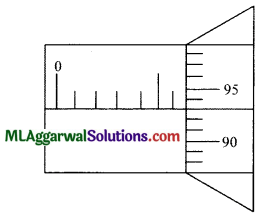(c) A simple pendulum is set up in a laboratory at the sea level. How would its time period change if it is shifted to :
(i) The moon,
(ii) a deep mine,
(iii) a place at Mount Everest
(iv) an artificial satellite on earth?
(a) (i) Micrometer screw gauge
(ii) Micrometer screw gauge
(iii) Vernier callipers

(b) $$\frac{\text { Pitch }}{\text { Number of divisions on circular scale }}$$
$$\frac{0.1}{100}$$ = 0.001cm
Reading of the screw gauge = MSR + CSD x LC
= 0.6 cm + 93 x 0.001 cm
= 0.6 + 0.093 = 0.693 cm.(c) (i) Time period increases = $$\left(\text { as } \mathrm{T} \propto \frac{1}{\sqrt{g}}\right)$$
(ii) Time period increases = $$\left(\text { as } \mathrm{T} \propto \frac{1}{\sqrt{g}}\right)$$
(iii) Time period increases as on a mountain, acceleration due to gravity decreases and  $$\mathrm{T} \propto \frac{1}{\sqrt{g}}$$
(iv) Time period becomes infinite, as in an artificial satellite, there is zero gravity and $$\mathrm{T} \propto \frac{1}{\sqrt{g}}$$

Question 6.
(a) A body P has a mass of 20 kg and is moving with a velocity of 5 m/s. Another body, Q has a mass of 5 kg and is moving with a Velocity of 20 m/s. Calculate the ratio of the momentum of P and
(b) What force would be required to accelerate a body of mass 20 kg from 1.5 ms-1 to 9 ms-1 in one-third of a minute ?
(c) In the given figure, the displacement of an object is shown at different times. Calculate the speed of the object as it moves for 0 s to 5 s, 5 s to 7 s, 7 s and 9 s. Also, calculate the average velocity during the time interval 5 s to 9 s.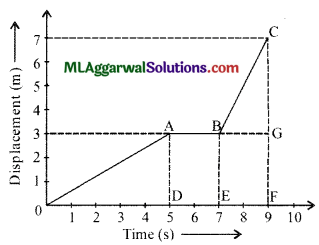(a) For body P,
Given : m = 20 kg, ν = 20 m/s
∴ Momentum of the body P = 20 kg x 5 m/s = 100 kg m/s.
For body Q,
Given : m = 5 kg, ν = 20 m/s
∴ Momentum of the body Q = 5 kg x 20 m/s = 100 kg m/s
$$\frac{\text { Momentum of body } \mathrm{P}}{\text { Momentum of body } \mathrm{Q}}=\frac{100}{100}=\frac{1}{1} \text { or } 1: 1$$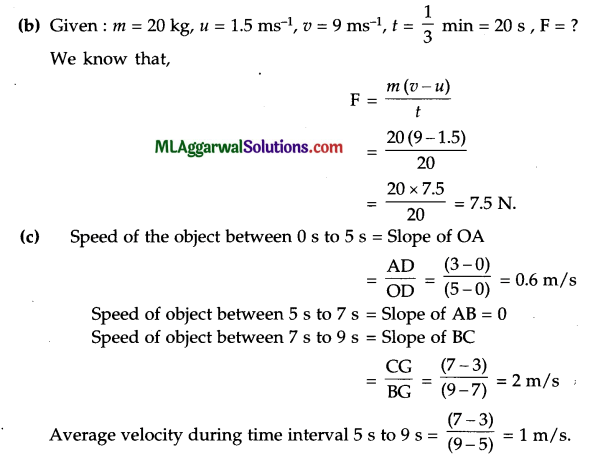Question 7.
(a) (i) Define pressure and give its S.I. unit.
(ii) The pressure in a water pipe on the ground floor of a building is 100000 Pa. What is the pressure in a water pipe on the first floor at a height of 2.5 m ? (Density of water = 1000 kg m-3, g = 10 ms-2)

(b) A given solid weighs 0.100 kgf in air, 0.080 kgf in a liquid and 0.075 kgf in water. What is : (i) the apparent loss of weight of the solid in liquid, (ii) the apparent loss of weight of the solid in water and (iii) the R.D. of the liquid ?

(c) A block of wood of density 0.8 x 103 kg/m3 just begins to sink when a mass of 1.6 kg is placed on it. What is the volume of the block ?
(i) The pressure at a given point is the (normal) force acting there per unit area. The
S.I. unit of pressure is Nm-2 or pascal (Pa).

(ii) We have,
Pressure on ground floor = Pressure on first floor + Pressure due to a water column of height 2.5 m
100000 Pa = Pressure on first floor + (2.5 x 1000 x 10) Pa
∴ Pressure on first floor = 100000 – (2.5 x 1000 x 10) Pa
= 75000 Pa

(b) (i) Apparent loss of weight of the solid in liquid = (0.100 – 0.080) kgf = 0.020 kgf
(ii) Apparent loss of weight of the solid in water = (0.100 – 0.075) kgf = 0.025 kgf
$$\text { R.D. of liquid }=\frac{\text { Loss of weight in liquid }}{\text { Loss of weight in water }}=\frac{0.020}{0.025}=0.8$$

(c) Under given condition
Weight of water displaced = Weight of the block + Weight placed on the block and, Volume of water displaced = Volume of the block.
Let the volume of the block be V m3.
Then, Mass of water displaced = (V) x (1000)[∵ Density of water = 1000 kg/m3]
∴ Weight of water displaced, W3 = V x 1000 x 9.8
Mass of block = V x ρ                                 [ρ = density of block]
∴ Weight of block, W1 = V x ρ x g
= V x 0.8 x 103 x 9.8
weight placed on the block W2 = 1.6 x 9.8
∴ W1 + W2 = V x 0.8 x 103 x 9.8 + 1.6 x 9.8
Dividing both sides by 9.8 ms-2 and on solving, we get
1000 V = 800 V + 1.6
or  (1000 – 800) V = 1.6 kg
$$\mathrm{V}=\frac{1.6}{200}$$ = 8 × 10-3 m3

Question 8.
(a) The given diagram shows an object PQ placed in front of a plane mirror. Complete the ray diagram to show the formation of image by the plane mirror of the object PQ.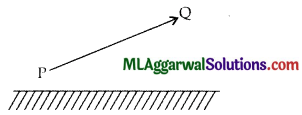(b) The angle between an incident ray and the mirror is θ as shown in the given figure. The total angle turned by the ray is 80°. What is the value of θ ?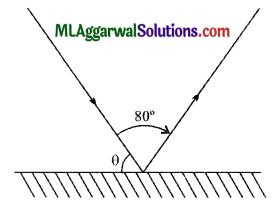(c) The diagram below shows a point object P in front of a plane mirror MN.
(i) Complete the diagram by taking two rays from the point P to show the formation of its image.
(ii) Is the image formed real or virtual ? Explain why.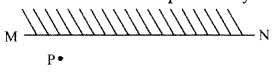(a) The completed ray diagram is as follows :(b) The ray diagram is redrawn as shown below :Since, Angle of incidence = Angle of reflection
Therefore, 9 = (90 – 40)° = 50°.

(c) (i) The ray diagram is as follows :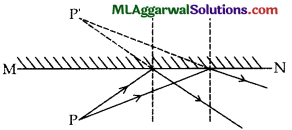(ii) The image formed is virtual because the reflected rays seem to meet behind the mirror.

Question 9.
(a) (i) The frequency of a source of sound is 100 Hz. How many times does it vibrate in a minute ?
(ii) People living near the railway tracks often press their ears against the track to find whether a train in coming or not. Why ?

(i) What causes sound ?
(ii) Can sound travel in vacuum ?
(iii) How does the speed of sound differ in different media ?

(c) The speed of sound in air is 340 ms-1 and in water is 1450 ms-1. It takes 2 s for sound to reach a certain distance from the source placed in air.
(i) Find the distance.
(ii) How much time will it take for sound to reach the same distance when the source is in water ?
(i) Number of vibrations produced in 1 s = 100
∴ Number of vibrations produced in 60 s = 100 x 60 = 6000

(iii) The reason is that the vibrations produced by the moving wheels of the train travel much faster through the steel track than through the air. So, the sound is heard through the track much before it is heard through the air. Thus, the person gets an indication of the coming of the train.

b(i) Sound is caused due to vibrations of a body.
(ii) No, sound cannot travel in vacuum.
(iii) Speed of sound is maximum in solids, less in liquids and least in gases.(c) (i) Given : In air, v = 340 ms-1, t – 2 s
From relation, $$v=\frac{d}{t}$$
Distance travelled by sound in air,
d = ν x t = 340 x 2 = 680 m.
ν = 1450 ms-1, d = 680 m

(ii) In water,
∴ Time taken by sound to travel the distance ‘d’ in water will be
$$t=\frac{d}{v}=\frac{680}{1450}=0.47 \mathrm{~s}$$

Question 10.
(a) The figure shows a coil wound around a soft iron bar XY.
(i) State the polarity at the ends X and Y as the switch is pressed.
(ii) Suggest one way of increasing the strength of electromagnet so formed.(b) A coil of insulated copper wire is wound around a piece of soft iron and current is passed in the coil from a battery.
(i) What name is given to the device so obtained?
(ii) Give two uses of the device mentioned by you.

(c) Name the physical quantities having the following S.I. units :
(i) volt
(ii) coulomb
(iii) ohm and
(iv) ampere.
(a) (i) X is north pole, Y is the south pole.
(ii) The strength of the electromagnet can be increased by increasing the current in the coil.(b) (i) The device obtained is an electromagnet.
(ii) An electromagnet can be used for the following purposes :
(1) For removing pieces of iron from wounds.
(2) In electrical devices like bell, telegraph, etc.

(c) (i) Potential difference
(ii) Charge